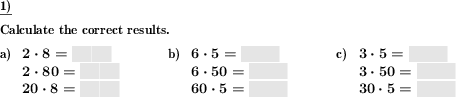Custom math worksheets at your fingertips# Details for problem "Times table with factor ten"

Quickname: 6733

Elementary School, Primary School, Middle School.

## Summary

Multiplication problem series introducing factor of ten

## Example## Description

This problem type varies simple multiplication problems from the times table by increasing factors tenfold.

Taking a product from the times table in the form of p = f1 * f2 as a baseline, three problems are derived by increasing one of the factors by an order of magnitude:

• f1 x f2 = for example, 7 x 6 =
• f1 x 10f2 = for example, 7 x 60 =
• 10f1 x f2 = for example, 70 x 6 =

This series may always appear in this order in a problem, in reverse order or in a random order.

There is an option to include a catch.

If chosen, one of the problems may contain a trick question in the form of a product that will contain a factor that does not follow this scheme but be a different one, for example the series may look like 7 x 3, 7 x 30, 50 x 3.

Download free printable worksheets for this math problem here. The worksheet contains the problems only, the solution sheet includes the answers. Just click on the respective link.

•Worksheet 1Solution sheet with answers
•Worksheet 2Solution sheet with answers
•Worksheet 3Solution sheet with answers

If you can not see the solution sheets for download, they may be filtered out by an ad blocker that you may have installed. If this is the case, please allow ads for this page and reload the page. The solution sheets will then reappear.

• Do these sample worksheets do not really fit?
• Do you need more math worksheets, with a different level of difficulty?
• Would you like to combine different problems on a worksheet and adjust them to your needs?
• As a teacher, you can put together your own worksheets using the automatically generated math problems provided.
With a free initial credit, you can start creating your own math worksheets in a few minutes.

You can try it for free! Register here, to create custom worksheets now!

## Customization options for this problem

Parameter
Possible values
Number of problems
1, 2, 3, 4, 5, 6, 7, 8, 9, 10
Order of product terms
random, ascending, descending
Insert trick question
Yes, No

## Similar problems

Remark
Description
With a series of division problems
Division problem series with divisor and dividend alternating with factor ten.
Simple multiplication problems in the times table range
Multiplication problems from the 1-10 times tables.